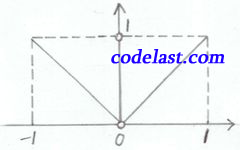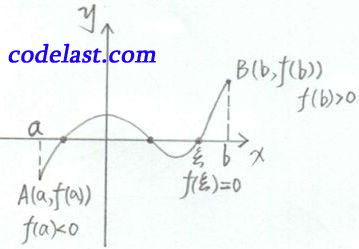# [原创]高等数学笔记(21)

【前言】

【正文】

$\mathop {\min }\limits_{x \in I} f(x) = f({x_0})$ （或 $\mathop {\max }\limits_{x \in I} f(x) = f({x_0})$

1.最大、最小值定理

$\mathop {\min }\limits_{x \in [a,b]} f(x) = f(\xi ),\;\mathop {\max }\limits_{x \in [a,b]} f(x) = f(\eta )$

$f(x) = \left\{ {\begin{array}{*{20}{c}}{ - x,}\\{1,}\\{x,}\end{array}}\right.\begin{array}{*{20}{c}}{ - 1 \le x < 0}\\{x = 0}\\{0 < x \le 1}\end{array}$$f(x)$$[ - 1,1]$ 上的 $x = 0$ 点处不连续， $f(x)$$[ - 1,1]$ 内无最小值。

2.有界性定理

3.零值点定理

$f(x)$$[a,b]$ 上连续，且 $f(a)$$f(b)$ 异号（即 $f(a)f(b) < 0$ ），则至少存在一点 $\xi$ ，使 $f(\xi ) = 0$

$y = f(x)$$[a,b]$ 上连续，则函数曲线 $y = f(x)$ 是连续曲线，两端点为 $A(a,f(a)),B(b,f(b))$4.介值定理
$f(x) \in C\left[ {a,b} \right]$ （即在 $[a,b]$ 上连续），且 $f(a) = A,f(b) = B,A \ne B$ ，则对于数 $C$$C$ 介于 $A,B$ 之间），则至少存在一点 $\xi$ ，使 $f(\xi ) = C$

$F(a) = f(a) - C = A - C < 0$
$F(b) = f(b) - C = B - C > 0$

$[{x_1},{x_2}]$$[{x_2},{x_1}]$ 上利用性质4即得结论。

$c = \min \{ {x_1},{x_2}, \cdots ,{x_n}\} ,\;d = \max \{ {x_1},{x_2}, \cdots ,{x_n}\}$
$[c,d] \subset (a,b)$ ，且 $f(x) \in C\left[ {c,d} \right]$

n个不等式相加：
$nm \le f({x_1}) + f({x_2}) + \cdots + f({x_n}) \le nM$
$m \le \frac{{f({x_1}) + f({x_2}) + \cdots + f({x_n})}}{n} \le M$

（第21课完）

➤➤ 版权声明 ➤➤